Function Repository Resource:

# RandomVector

Return a pseudorandom vector of a given type and size

Contributed by: Dennis M Schneider
 ResourceFunction["RandomVector"][type,range,n] returns an n-dimensional vector with entries that are random values of type type and lie in range range.

## Details

The type can be Integer, Rational, Real, Complex, "GaussianInteger" or "GaussianRational".
For complex types, the range is given as a pair of complex values that are taken as diagonally opposite endpoints of a rectangle.

## Examples

### Basic Examples (7)

A random three-dimensional vector whose entries are approximate real numbers between 0 and 1:

 In:=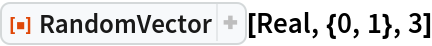Out=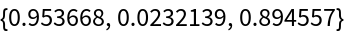A random three-dimensional vector whose entries are approximate real numbers between -5 and 10:

 In:=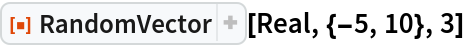Out=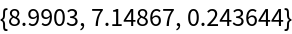A random three-dimensional vector whose entries are integers between -5 and 10:

 In:=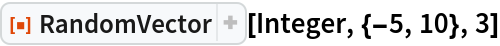Out=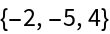A random three-dimensional vector whose entries are rationals between -5 and 10:

 In:=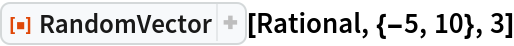Out=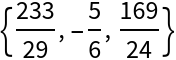A random three-dimensional vector whose entries are Gaussian integers between -5 and 10:

 In:=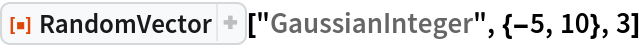Out=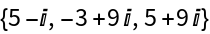A random three-dimensional vector whose entries are Gaussian rationals between -5 and 10:

 In:=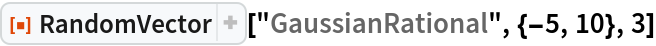Out=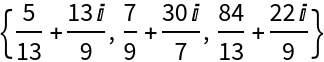A random three-dimensional vector whose entries are RandomComplex[{-5+2I,10-3I}]:

 In:=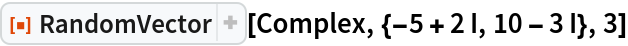Out=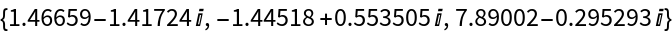## Publisher

Dennis M Schneider

## Version History

• 1.0.0 – 20 January 2021